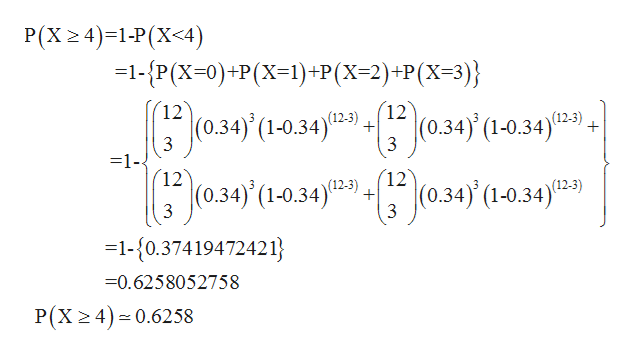# 34% adults favor the use of unmanned drones by police agencies. Twelve U.S. adults are randomly selected. Find the probability that the number of U.S. adults who favor the use of unmanned drones by police agencies is​ (a) exactly​ three, (b) at least​ four, (c) less than eight.

Question
20 views
34% adults favor the use of unmanned drones by police agencies. Twelve U.S. adults are randomly selected. Find the probability that the number of U.S. adults who favor the use of unmanned drones by police agencies is​ (a) exactly​ three, (b) at least​ four, (c) less than eight.
check_circle

Step 1

From the given information,

Binomial parameters, n = 12 and P=34% .034.

Here we can use binomial distribution probability.

Let ‘X’ be a binomial random variable.

Formula for binomial probability is,

Step 2

Now,

(a) P(x=3) is,

Step 3

(b) P (at least...help_outlineImage TranscriptioncloseP(X 2 4) 1-P (X<4) -1-P(X-0)+P(X1)+P(X=2)+P(X=3)} 12 12 3 0.34) (1-0.34)09 (0.34) (1-0.34)(2) 3 1- 12 12 (0.34) (1-0.34)09 (0.34)' (1-0.34)2) 3 3 -1-{0.37419472421 =0.6258052758 P(X 2 4) 0.6258 fullscreen

### Want to see the full answer?

See Solution

#### Want to see this answer and more?

Solutions are written by subject experts who are available 24/7. Questions are typically answered within 1 hour.*

See Solution
*Response times may vary by subject and question.
Tagged in

### Statistics